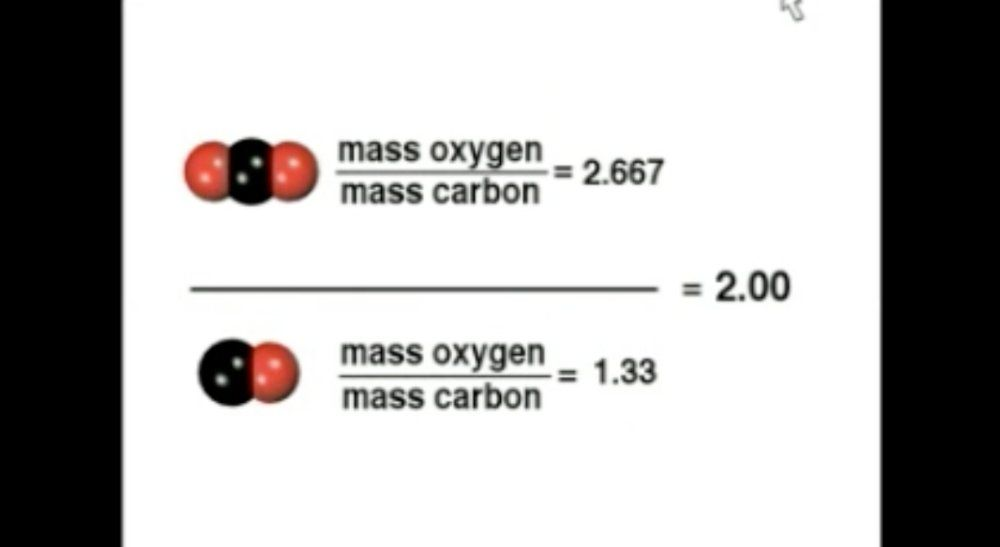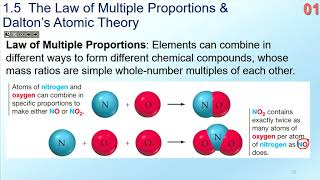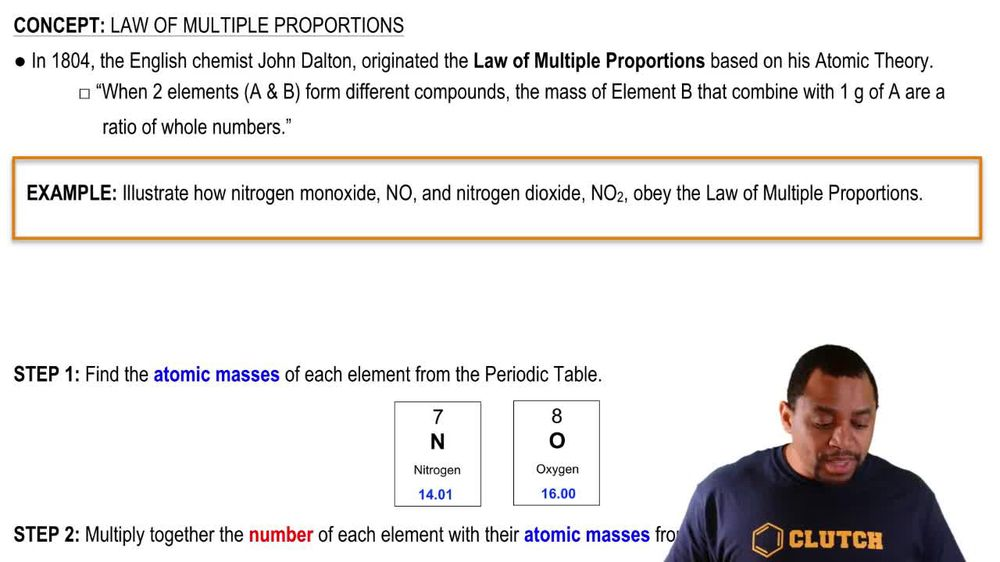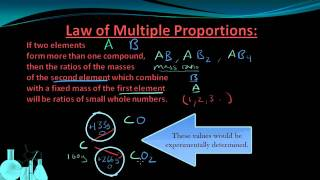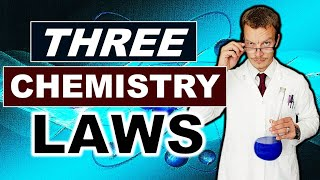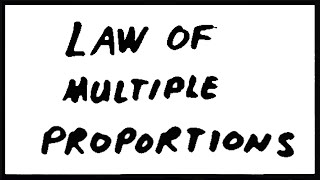Start typing, then use the up and down arrows to select an option from the list.
1. 2. Atoms & Elements2. Law of Multiple Proportions
Problem

# The atomic weight of carbon (12.011) is approximately 12 times that of hydrogen (1.008). (a) Show how you can use this knowledge to calculate pos-sible formulas for benzene, ethane, and ethylene (Prob-lem 2.82).

Relevant Solution6m
Play a video:
Hello everyone today we are being given the falling problem. Nitrogen with an atomic mass of 14.007 has an atomic weight. That is roughly 14 times more than hydrogen, which is 1.8 atomic mass units. Using the following day to give the potential formulas for dai assign try a zine and hexes. So we're going to separate them. We're going to do design up here, try isn't over here and last but not least Alex cuisine. And so we're going to do a series of calculations for this problem. For example, we're going to take the mass. So for example, the mass of *** identifying the nitrogen atoms or how many would be 2.62 g times the conversion factor that one atomic mass unit is equal to 1.66 times 10 to the negative 24 g. To find out how many g we have. We then use the conversion factor that one nitrogen atom has 14.007g. Once our units cancel out, we end up with 1.13 times 10 To the 23rd nitrogen atoms. And we then do the same for the mass of hydrogen atoms. So you have 0.38 grams. We use the conversion factor of one atomic mass unit equals 1.66 times 10 to the negative 24 g. And then we also use the conversion factor that one hydrogen atom according to the periodic table equal to 1.8 g onions, canceled handling it up with 2.27 times 10 to the 23rd hydrogen atoms. And so now lastly for this, we have to find a possible formula for diocese. And so what we're gonna do is we're going to take the ratio of nitrogen atoms two hydrogen atoms and divide them. So for nitrogen, what we solved for is 1.13 times 10 to the 23rd Over our 2.27 times 10 to the 23rd hydrogen atoms. And this yields us roughly one nitrogen for every two hydrogen and so possible formula for dia zine is N H two. So that's going to be the answer for our first part. Now we can move on to try a scene. We're going to use the same method, meaning we're going to take 2.8 grams. Multiplied by the conversion factor that one atomic mass unit is equal to 1.66 times 10 to the negative 24 g times a conversion factor that one nitrogen atom is equal to 14.7 g. And when units cancel that were left with 1.23 to times 10 to the 23rd nitrogen atoms. And I do this everything for the point of 20 g, We get 1.19 times 10 to the 23rd nitrogen atoms. As before. We're going to find the ratio of nitrogen atoms two hydrogen atoms with the following equation. We plug in our value for nitrogen, atoms which is 1.2 times 10 to the 23 over our hydrogen. That should be an H. for our hydrogen which should be 1.19 times 10 to the 23rd. And we get approximately one nitrogen per one hydrogen, meaning that a possible formula Fortress scene is N. H. And so that's solving the second part. Last but not least we installed. We must solve for hex hex Zane. And so we follow the same principle. We take our masks for nitrogen 2.74 g and we multiplied by the conversion factor that one atomic mass unit is equal to 1.66 times to the negative 24 g. And then that one nitrogen atom Is equal to 14.007 g. This yields us 1.178 times 10 to the 23rd nitrogen atoms. And then lastly we take our 0.26 g times one atomic mass unit over 1.66 times 10 to the negative 24 g. And then lastly, we do our one nitrogen when hydrogen is equal to 1. grams. Doing that, we get 1.55 times 10 to the 23rd hydrogen atoms. And then our last equation here will be the ratio between nitrogen atoms and hydrogen atoms. With nitrogen of course being the 1.178 times 10 to the 23rd nitrogen atoms over our 1. times 10 to the 23rd hydrogen atoms. And we get approximately three nitrogen for every four hydrogen. So possible formula for hex sign could be N three H four. And with that we have solved the problem overall, I hope this helped until next time.